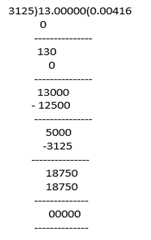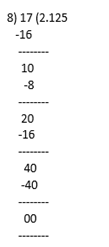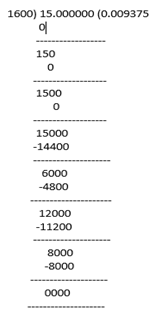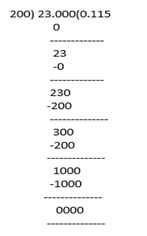# Chapter 1 Real Numbers

At Saralstudy, we are providing you with the solution of Class 10 Mathematics Real Numbers according to the latest NCERT (CBSE) Book guidelines prepared by expert teachers. Here we are trying to give you a detailed answer to the questions of the entire topic of this chapter so that you can get more marks in your examinations by preparing the answers based on this lesson. We are trying our best to give you detailed answers to all the questions of all the topics of Class 10th mathematics Real Numbers so that you can prepare for the exam according to your own pace and your speed.

Download pdf of NCERT Solutions for Class Mathematics Chapter 1 Real Numbers

Download pdf of NCERT Examplar with Solutions for Class Mathematics Chapter 1 Real Numbers

### Exercise 1 ( Page No. : 7 )

•  Q1 Use Euclid’s division algorithm to find the HCF of :    (i) 135 and 225      (ii) 196 and 38220      (iii) 867 and 255 Ans: (i) Here, we have to find H.C.F of 135 and 225 First divide divide the larger integer smaller integer Since, 225 > 135 Therefore, by Euclid’s Division algorithm 225 = 135 × 1 + 90                                       (i) Here 90 ≠ 0, so proceed the same procedure further Again by E.D.L,              (E.D.L = Euclid’s division algorithm) 135 = 90 × 1 + 45                                         (ii) As we know, 45 ≠ 0 therefore, again by E.D.L 90 = 45 × 2 + 0                                             (iii) Here, r = 0 so we cannot proceed further. The divisor at this Stage is 45. From (i), (ii) and (iii) H.C.F (225, 135) = H.C.F (135, 90) = H.C.F (90, 45) = 45.   (ii) Here, we have to find H.C.F of 38220 and 196 First divide the larger integer smaller integer Since, 3822 > 196 Therefore by Euclid’s Division Algorithm 38220 = 196 × 195 + 0 Here, r = 0 so we cannot proceed further. The divisor at this Stage is 196. Hence, H.C.F (38220, 196) = 196.   (iii) Here, we have to find H.C.F of 867 and 255 First divide the larger integer smaller integer Since, 867 > 255 Therefore, by Euclid’s Division algorithm 867 = 255 × 3 + 102                           (i) Remainder 102 ≠ 0, so proceed the same procedure further using E.D.L 255 = 102 × 2 + 51                            (ii) Here, 51 ≠ 0 again using E.D.L = 51 × 2 102 = 51 × 2 + 0                                (iii) Here, r = 0 so we cannot proceed further. The divisor at this Stage is 51. From (i), (ii) and (iii) H.C.F (867, 255) = H.C.F (255, 102) = H.C.F (102, 51) = 51. Q2 Show that any positive odd integer is of the form 6q + 1, or 6q + 3, or 6q + 5, where q is some integer. Ans: Let a be any positive integer b = 6. Then by Euclid’s algorithm, a = 6q + r for some integer q ≥ 0 So, values of r we get, r = 0, 1, 2, 3, 4, 5 When, r = 0, then, a = 6q + 0, similarly for r = 1, 2, 3, 4, 5 the value of a is 6q+1, 6q+2, 6q+3, 6q+4 and 6q+5 respectively. If a = 6q, 6q+2, 6q+4, then a is even and divisible by 2. Therefore, any positive odd integer is in the form of 6q+1, 6q+3, 6q+5 Where q is some integer. Q3 An army contingent of 616 members is to march behind an army band of 32 members in a parade. The two groups are to march in the same number of columns. What is the maximum number of columns in which they can march? Ans: Total no. of army contingent members = 616 No. of army band members = 32 To find max. numbers of the same columns in which the both groups march. We have to find it. Their highest common factor. since, 616 > 32 then, by Euclid’s algorithm 616 = 32 × 19 + 8                            (i) Here 8 ≠ 0 then by again using Euclid algorithm 32  =  8 × 4 + 0                                (ii) Here, r =0 so we cannot proceed further. The divisor at this Stage is 8. So the no. of columns is 8. Q4 Use Euclid’s division lemma to show that the square of any positive integer is either of the form 3m or 3m + 1 for some integer m. [Hint : Let x be any positive integer then it is of the form 3q, 3q + 1 or 3q + 2. Now square each of these and show that they can be rewritten in the form 3m or 3m + 1.] Ans: Let x be any positive integer and b = 3. Then, by euclid’s algorithm x = 3q + r, where q ≥ 0 and r = 0, 1, 2          [0 ≤ r ≤ b] Case (i) :    For r = 0, x = 3q, = x2 = 9q2, taking 3 as common,                    x2 = 9q2  = 3 (3q2), which is of the form 3m, where m = 3q2. Case (ii) :   For r = 1, x = 3q + 1                    x2 = 9q2 + 1 + 6q, taking 3 as common,                    = 3 (3q2 + 2q) + 1, which is of the form 3m + 1, where m = 3q2 + 2q Case (iii) :   For r = 2, 3q + 2                    x2 = 9q2 + 4 + 12q = (9q2 + 12q + 3) + 1, taking 3 as common,                    = 3 (3q2 + 4q + 1) + 1, which is of the form 3m +1, where m = 3q2 + 4q + 1                    Hence, x2 is either of the form 3m, 3m + 1 for some integer m. Q5 Use Euclid’s division lemma to show that the cube of any positive integer is of the form 9m, 9m + 1 or 9m + 8. Ans: Let a be any positive integer and b = 3. Then, by euclid’s algorithm a = 9q + r, where q ≥ 0 and r = 0, 1, 2                                         [ 0 ≤ r ≤ b ] For r = 0, x = 3q,   or For r = 1, x = 3q +1 For r = 2, x = 3q + 2 Now by taking the cube of all the three above terms, we get, Case (i) :      when r = 0, then,                      x3 = (3q)3 = 27q3  = 9 (3q3) = 9m; where m = 3q Case (ii) :     when  r = 1, then,                      x3 = (3q +1)3 = (3q)3 + 13 +3 × 3q × 1 (3q + 2 ) = 27q3 + 1 +27q2 + 9q                      Taking 9 as common factor, we get,                      x3 = 9 (3q3 +3q2 + q) +1                      Putting  (3q3 + 3q2 + q) = m, we get,                      x3 = 9m + 1 Case (iii) :     when r = 2, then,                      x3 = (3q + 2)3 = (3q)3 + 23 + 3 × 3q × 2 (3q + 2) = 27q3 + 54q2 + 36q + 8                      Taking 9 as common factor , we get                       x = 9 (3q + 6q + 4q) + 8                      Putting (3q + 6q + 4q) = m, we get,                      x = 9m + 8, Therefore, it is proved that the cube of any positive integer is of the form 9m, 9m + 1, 9m + 8.

### Exercise 2 ( Page No. : 11 )

•  Q1 Express each number as a product of its prime factors:    (i) 140     (ii) 156     (iii) 3825     (iv) 5005     (v) 7429 Ans: (i) 140  By taking the L.C.M of 140, we will get the product of its prime factors. Therefore, 140 = 2 × 2 × 5 × 7 ×1 = 22 × 5 × 7 (ii) 156 By taking the L.C.M of 156, we will get the product of its prime factors. Therefore, 156 = 2 ×  2 × 13 × 3 × 1 =  22 × 13 × 3 (iii) 3825 By taking the L.C.M of 3825, we will get the product of its prime factors. Therefore, 3825 = 3 × 3 × 5 × 5 × 17 × 1 = 32 × 52 × 17 × 1 (iv) 5005  By taking the L.C.M of 5005, we will get the product of its prime factors. 5005 = 5 × 5 × 11 × 13 × 1 = 5 × 7 × 11 × 1 (v) 7429  By taking the L.C.M of 7429, we will get the product of its prime factors. Therefore, 7429 = 17 × 19 × 13 × 1 Q2 Find the LCM and HCF of the following pairs of integers and verify that LCM × HCF = product of the two numbers.    (i) 26 and 91     (ii) 510 and 92     (iii) 336 and 54 Ans: (i) 26 and 91 First we have to find the L.C.M and H.C.F of 26, 91 using fundamental theorem of arithmetic, 26 = 13 × 2 91 = 13 × 7 For L.C.M - list all the prime factors (only once) of 26, 91 with their greatest power. L.C.M (26, 91) = 13 × 2 × 7 = 182 For H.C.F – write all the common factors (only once) with their smallest exponent. H.C.F (26, 91) = 13 Verification :   L.C.M (26, 91) × H.C.F (26, 91) = 26 × 91                       182 × 13 = 2366,       => 2366 = 2366                       Hence, proved. (ii) 510 and 92 First we have to find the L.C.M and H.C.F of 510, 92 using fundamental theorem of arithmetic, 510 = 2 × 3 × 5 × 17 92 = 2 × 2 × 23 For L.C.M - list all the prime factors (only once) of 510, 92 with their greatest power. L.C.M (510, 92) = 2 × 2 × 3 × 5 × 17 × 23 = 23460 For H.C.F – write all the common factors (only once) with their smallest exponent. H.C.F (510, 92) = 2 Verification :  L.C.M (510, 92) × H.C.F (510, 92) = 510 × 92                      23460 × 2 = 46920,                 =>  46920 = 46920                      Hence, proved. (iii) 336 and 54 First we have to find the L.C.M and H.C.F of 336, 54 using fundamental theorem of arithmetic, 336 = 2 × 2 × 2 × 2  × 3 × 7 = 24 × 3 × 7 54 = 2 × 3 × 3 × 3 = 2 × 33 For L.C.M - list all the prime factors (only once) of 336, 54 with their greatest power. L.C.M (336, 54) = 3024 For H.C.F – write all the common factors (only once) with their smallest exponent. H.C.F (336, 54) = 2 × 3 = 6 Verification : L.C.M (336, 54) × H.C.F (336, 54) = 336 × 54                      3024 × 6 = 18144                    => 18144 = 18144                      Hence, proved. Q3 Find the LCM and HCF of the following integers by applying the prime factorisation method.    (i) 12, 15 and 21     (ii) 17, 23 and 29     (iii) 8, 9 and 25 Ans: (i) 12, 15 and 21 12 = 2 × 3 × 2 15 = 3 × 5 21 = 3 × 7 L.C.M (12, 15, 21) = 2 × 3 × 2 × 5 × 7 = 420 H.C.F (12, 15, 21) = 3   (ii) 17, 23 and 29 17 = 17 × 1 23 = 23 × 1 29 = 29 ×1 L.C.M (17, 23, 29) = 11339 H.C.F (17, 23, 29) = 1 (iii) 8, 9 and 25 8 = 2 × 2 × 2 × 1 9 = 3 × 3 × 1 25 = 5 × 5 × 1 L.C.M (8, 9, 25) = 1800 H.C.F (8, 9, 25) = 1 Q4 Given that HCF (306, 657) = 9, find LCM (306, 657). Ans: Given, H.C.F (306, 657) = 9 First number (a) = 306 Second number (b) = 657 As we know, H.C.F × L.C.M = a × b 9 × L.C.M = 306 × 657 L.C.M = (306 × 657) / 9 = 22338 Hence, L.C.M (306, 657) = 22338 Q5 Check whether 6n can end with the digit 0 for any natural number n. Ans: Suppose the number 6n for any n € N ends with zero. In this case 6n is divisible by 5. Prime factorization of 6n = (2 × 3)n Therefore, the prime factorization of 6n doesn’t contain prime number 5. Hence, it is clear that for any natural number n, 6n is not divisible by 5 and thus it proves that 6n cannot end with the digit 0 for any natural number n. Q6 Explain why 7 × 11 × 13 + 13 and 7 × 6 × 5 × 4 × 3 × 2 × 1 + 5 are composite numbers. Ans: Composite numbers are factors other than 1 and itself. From here we can observe that : (a) 7 × 11 × 13 + 13 = 13 (7 × 11 × 1 + 1) = 13 (77 + 1)                = 13 × 78                = 13 × 13  × 6 Therefore, this expression has 6 and 13 as its factors. Hence, it is a composite number. (b) 7 × 6 × 5 × 4 × 3 × 2 × 1 + 5 = 5 (7 × 6 × 1 × 4 × 3 × 2 × 1 + 1)                                                   = 5 × (1008 + 1)                                                   = 5 × 1009 1009 can not be factored further. Therefore, this expression has 5 and 1009 as its factors. Hence, it is a composite number. Q7 There is a circular path around a sports field. Sonia takes 18 minutes to drive one round of the field, while Ravi takes 12 minutes for the same. Suppose they both start at the same point and at the same time, and go in the same direction. After how many minutes will they meet again at the starting point? Ans: Time taken by Sonia to drive one round = 18 min Time taken by Ravi to drive one round = 15 min Let they both meet after time ‘t’. Here, ‘t’ is L.C.M of 18, 12 Now, 18 = 2 × 3 × 3 12 = 2 × 3 × 2 L.C.M (18, 12) = 22 × 32 = 36 Thus, they both meet after 36 minutes at the starting point.

### Exercise 3 ( Page No. : 14 )

•  Q1 Prove that √5 is irrational. Ans: Let us consider √5 is a rational number. Therefore, √5 = pq, where p and q are integers and q ≠ 0 If pa and q have any common factor ,then dividing by that common factor, We have, √5 = ab, where a and b are co primes. a = √5b On squaring, a² = 5b²                ...........   (1) Due to the presence of 5 on RHS, we say that 5 is a factor of a². 5 divides a² Since, a is prime , therefore 5 divides a                                   [ theorem ] Therefore , a = 5k , where k is an integer. Putting a = 5k in (1) , we have 25k² = 5b²  b² = 5k² This shows that 5divides b². But b is a prime no and so 5 divides b also. Thus 5 is a common factor of a and b . This is contradiction to the fact that a and b have no common factor other than one. Thus our consideration is wrong and so √ 5 is not a rational number. Hence,  proved. Q2 Prove that 3 + 2√5 is irrational. Ans: Let us assume 3 + 2√5 is a rational number. Therefore, 3 + 2√5 = p/q where p and q are co primes and q ≠ 0. 3 + 2√5 = ab            On solving, 2√5 =(a/b) - 3 √5 =1/2 (a/b - 3) Since a, b are integers and 1/2 (a/b-3 ) is also a rational number. But we know √5 is an irrational number. Thus our assumption is wrong. 3 + 2√5 is not a rational number. Hence proved. Q3 Prove that the following are irrationals: Ans: (i) Let us assume 1/√2 is a rational number. 1/√2 = p/q , where q ≠ 0 and p and q are co primes. On reciprocal, √2 = qp                                 ................(1) Since, q and p are integers and q/p is also a rational number As we know √2 is an irrational number. From (1)  √2 ≠ q/p Thus our assumption is wrong 1/√2 is not a rational number. Hence, proved    (ii) Let us suppose 7√5 is a rational number. 7√5 = p/q, where p and q are co primes and q ≠ 0 On solving , √5 = (p/q)7                .....................(1) Since p, q and 7 integers and (p/q)7 is also a rational number. And we know √5 is an irrational number. From (1)  √5 ≠ (p/q) / 7 So our supposition Is wrong 7√5 is not a rational number. Hence, proved.   (iii) Let us suppose 6 + √2 is a rational number. 6 + √2 = a/b, where a, b are co primes and b ≠ 0. On solving, √2 = a/b - 6                                  .....................(1) Since a, b and 6 are integers and a/b - 6 is also a rational number. And we know that √2 is an irrational number. From (1) √2 ≠ a/b - 6 Thus our Superposition is wrong 6√2 is not a rational number. Hence, proved.

### Exercise 4 ( Page No. : 18 )

•  Q1 Without actually performing the long division, state whether the following rational numbers will have a terminating decimal expansion or a non-terminating repeating decimal expansion: Ans: Note: - If x be a rational number, then x can be expressed in the form p/q where p and q are Co- primes. Then, if the prime factorization of denominator (q) is in the form of 2m × 5n where n, m are non- negative integers, then x has a decimal expansion which terminates. If the prime factorization is not in this form of 2m × 5n then x has a decimal expansion which is non terminating. (i)                  13 / 3125      = 5 × 5 × 5 × 5 × 5 = 5⁵ (on factorization of q)     Since, its factorization contains only power of 5.     Therefore, it has a terminating decimal expansion. (ii)                 17 / 5                           = 2 × 2 × 2 = 2³                                        (on factorization of q)                       Since, its factorization contains only power of 2. Therefore, it has a terminating decimal expansion. (iii)                  64 / 455   = 5 × 7 × 13 = 5¹ × 7 × 13                           (on factorization of q)                       Since the factorization of not in the 2m ×5n                      Therefore, it has a non -terminating decimal expansion.   (iv)                  15 / 1600                         = 2 × 2 × 2 × 2 × 2 × 2 × 5 × 5 = 2⁶ × 5²          (on factorization of q)                       Since the factorization of q is in the form 2m ×5n                       Therefore, it has a terminating decimal expansion   (V)                 29 / 343                                                          = 7 × 7 × 7 = 7³                                         (on factorization of q)                        Since the factorization of denominator is not in the form 2m × 5n                        Therefore it has a non -terminating repeating decimal  (vi)                    23 / 23 52                          Since factorization of q already given and it is in the form 2m × 5n                      Therefore it has a terminating decimal expansion. (vii)               129 / 22 57 75                        Since factorization of q already given but it is not in the form 2m × 5n                      Therefore it has a terminating decimal expansion. (viii)                6 / 15 = 2/5                        5 = 5 × 1                                                (on factorization of q)                       Since 5 is the only factor in denominator.                                          Therefore it has a non -terminating repeating decimal expansion. (ix).                35 / 50 = 7 / 10                        10 = 2 × 5 = 2¹ × 5                                (on factorization of q)                       Since the factorization of denominator is in the form 2m × 5n                       Therefore it has a terminating decimal expansion. (x)                 77 / 210 = 11/30                         30 = 2 × 3 × 5 × 7 = 2¹ × 3 × 5 × 7.            (on factorization of q)                      Since the factorization of denominator is not in the form 2m × 5n                     Therefore it has a non -terminating repeating decimal expansion. Q2 Write down the decimal expansions of those rational numbers in Question 1 above which have terminating decimal expansions. Ans: (i)                  13 / 3125(ii)          17 / 8(iii)            64/455 is non terminating repeating decimal expansion. (iv)           15 / 1600(v)                 29/343 is non terminating repeating decimal expansion. (vi)                23 / 23 52(vii)              120 / 23 57 75 is non terminating repeating decimal expansion. (viii)               6/15 = 2/5Since, the given decimal expansion is non- terminating repeating. Therefore it is a rational number and we know that the prime factorization of denominator of rational 2 and 5 or both.